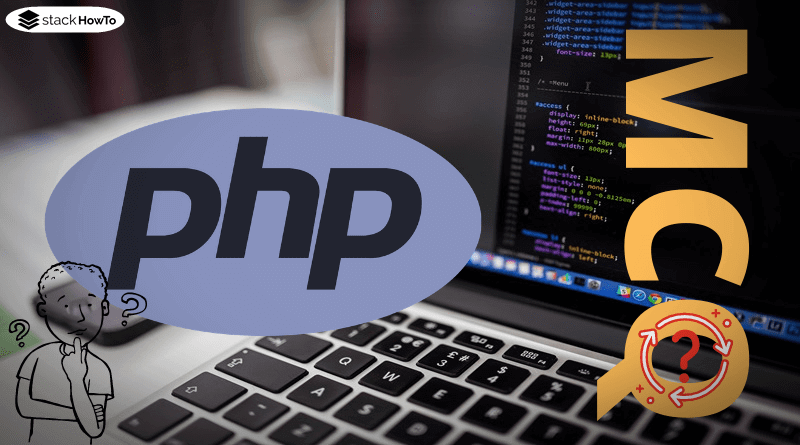php

# PHP Variable Scope

Which areas of application or scopes exist and what effects this has on access to variables?

According to the name, the scope of a variable is the area in which it is “active”. Variables cannot be read outside their scope. In PHP, the variable scope is typically formed by functions. Variables that are defined in the “body” of a function (ie the area between the curly brackets) are only valid in this area. When the function is terminated, the variables in their scope are automatically deleted.

The following example defines a variable \$x in the global scope. An attempt is made to access this variable using “myfunction()”. However, this access fails because the variable is outside the scope of the function. Then the variable is accessed within the “if” query. Since the “if” query is within the global scope of the function, this access is successful.

```<?php
\$x = 'example';

function myfunction() {
var_dump(\$x);
}

myfunction();

if (true) {
var_dump(\$x);
}
?>```

Output:

```Notice:  Undefined variable: x in [...][...] on line 5
NULL
string(7) "example"```PHP MCQ – Multiple Choice Questions and Answers – Basics – Part 1This collection of PHP Multiple Choice Questions and Answers (MCQs): Quizzes & Practice Tests with Answer focuses on “PHP basic”.   1. In PHP, variables…Read More
###### Example 2: Variables from a different scope cannot be overwritten

Since each scope is separate from the others, it is safe to use variable names more than once. There is no need to worry about accidental overwriting. In the next example, the variable \$x is assigned a value both in the global scope and in the scope of “myfunction()”. After leaving “myfunction()”, the local \$x variable is lost. The next time \$x is accessed in the global scope, the variable will therefore still contain the expected value 50 and not 500, which was assigned to it in the function.

```<?php
\$x = 50; // \$x in the global scope

function myfunction() {
// a local version of \$x is defined within myfunction()
\$x = 500;
var_dump(\$x);
}

myfunction();
?>```

Output:

```int(500)
int(50)```

###### Example 3: When leaving the scope, its variables are lost

If a variable is defined in a scope and no variable with the same name exists in the next higher scope, the value of the variable is still lost when leaving the scope of application. In the example, the variable \$x with the value 500 is defined in the function “myfunction()”. After leaving the function, an attempt is made to output the variable \$x. This fails because \$x is also lost when leaving “myfunction()” and no variable named “\$x” exists in the global scope.

```<?php
function myfunction() {
\$x = 500;
}

myfunction();
var_dump(\$x);
?>```

Output:

```Notice:  Undefined variable: x in [...][...] on line 7
NULL```PHP global variable with ExampleVariables usually have a scope, so variables defined in one function are not available for other functions. However, it is also possible to place variables…Read More

Variables that are in the global scope can be “imported” into a local scope. Either the keyword “global” or the array “\$GLOBALS” is used.

```<?php
\$x = 'example';

function myfunction() {
global \$x;  // Variable \$x from the global scope is now also
// valid in scope of the function myfunction()
var_dump(\$x);
}

myfunction();
?>```

Output:

`string(7) "example"`

###### Example 5: You can access superglobal variables from anywhere

A special category of variables are the superglobal variables. These variables are valid everywhere, so you can access them at any time, regardless of their current scope. Examples of superglobals are \$_GET (get parameters from the URL), \$_POST (usually form input), \$_SERVER (environment variables), \$_COOKIE (visitor cookies) and \$GLOBALS (global variables defined in the script).

```<?php
// a value is stored in the superglobal variable \$_GET
\$_GET['site'] = 'StackHowTo';

function myfunction() {
// This value can also be easily accessed in a function
var_dump(\$_GET['site']);
}

myfunction();
?>```

Output:

`string(10) "StackHowTo"`PHP – Static VariablesIn this tutorial, we’re going to see how to use the static variables in functions in PHP. If the scope of a function is abandoned,…Read More
###### Example 6: Nested functions form separate scopes

Functions can be defined nested within each other – but their scopes are not nested as well, but remain separate. The following example defines the function subFunction() within myfunction(). From subFunction() an attempt is now made to access a variable (\$y) defined in myfunction(), which generates an error. Then the value of \$y is output in myfunction().

```<?php
function myfunction()
{
\$y = 'example';
function subFunction()
{
var_dump(\$y);
}

subFunction();
var_dump(\$y);
}

myfunction();
?>```

Output:

```Notice:  Undefined variable: y in [...][...] on line 7
NULL
string(7) "example"```

###### Example 7: if and loops are not scopes

In many programming languages, a scope is introduced and ended by curly brackets. This is not the case in PHP. Only functions have their own scope (apart from the global scope). As a result, variables that are defined within if-else statements or loops are not lost when they end, but are valid beyond.

```<?php
// Example for if-else
if (true) {
\$a = 500;
}

var_dump(\$a);

// Example of loops
\$arr = array(1, 2, 3);

foreach (\$arr as \$value)
{
\$var = 'lorem';
echo(\$value . ", ");
}
echo("\n");

// these variables from foreach have "survived":
var_dump(\$var);
var_dump(\$value);
?>```

Output:

```int(500)
1, 2, 3,
string(5) "lorem"
int(3)```Type casting in PHPType Cast is the conversion of a variable of data type A into another data type B. For example, converting an integer variable into a…Read MoreMCQPractice competitive and technical Multiple Choice Questions and Answers (MCQs) with simple and logical explanations to prepare for tests and interviews.Read More